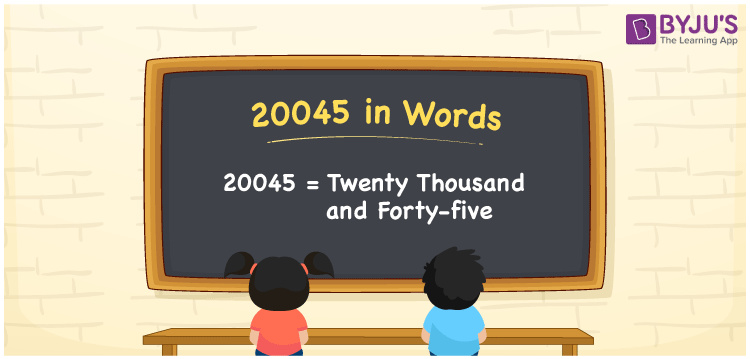# 20045 in Words

20045 in words is “Twenty thousand and forty-five”. The numeral 20045 is a natural number that comes after 20044 and 20046. The conversion of the number 20045 into words can be performed using the place value system. Also, 20045 is a cardinal number. For example, the cost of 20 books is Rs. 20045.

 20045 in Words: Twenty Thousand and Forty-five. Twenty Thousand and Forty-five in Numerical Form: 20045.

## 20045 in English Words## How to Write 20045 in Words?

Learn the place values of 20045 using the below table.

 Ten-thousands Thousands Hundreds Tens Ones 2 0 0 4 5

The expanded form of 20045 is as follows:

= 2 × Ten thousand + 0 × Thousand + 0 × Hundred + 4 × Ten + 5 × One

= 2 × 10000 + 0 × 1000 + 0 × 100 + 4 × 10 + 5 × 1

= 20000 + 40 + 5

= 20045

= Twenty thousand and forty-five

Hence, 20045 in words is twenty thousand and forty-five.

20045 in words – Twenty thousand and forty-five

Is 20045 an odd number? – Yes

Is 20045 an even number? – No

Is 20045 a perfect square number? – No

Is 20045 a perfect cube number? – No

Is 20045 a prime number? – No

Is 20045 a composite number? – Yes

## Frequently Asked Questions on 20045 in Words

Q1

### Write 20045 in words.

20045 in words is twenty thousand and forty-five.

Q2

### Simplify 20000 + 45, and express it in words.

Simplifying 20000 + 45, we get 20045. Hence, 20045 in words is twenty thousand and forty-five.

Q3

### Is 20045 a prime number?

No, 20045 is not a prime number.